# Time-Optimal Path Param.: a tool for Humanoid Motion Planning and Predictive ControlStéphane Caron
LIRMM, CNRS-UM2
Talk at Humanoids 2016, W3
November 15, 2016
https://scaron.info/slides/humanoids-2016/In this talk...# Time-Optimal Retiming

## Kino-dynamic process

1. Interpolate a path sq(s)
2. Find a path retiming ts(t)

## Disclaimer

It is not for time optimality that we use time-optimal retiming.# Manipulator

## Full dynamics

Equations of motion for a fixed-base manipulator:

M(q)¨q+˙qC(q)˙q+g(q)=τ

subject to torque constraints |τ|τmax.

## Path-tracking dynamics

The robot tracks the path sq(s) retimed as s(t):

˙q=qs˙s¨q=qs¨s+qss˙s2τ=m(s)¨s+c(s)˙s2+g(s)

Torque constraints can then be written as  a(s)¨s+b(s)˙s2+c(s)0.

# Time-optimal solutions

TOPP applies to all systems for which path-tracking constraints can be written:

a(s)¨s+b(s)˙s2+c(s)0

## Pontryagin's maximum principle

At each s(t) of the retimed trajectory:

• either ¨s is maximal: ¨s=β(s,˙s)
• or ¨s is minimal: ¨s=α(s,˙s)

We know how to enumerate switch points where the optimal solution alternates between α and β [Pham, TRO 2014].

# Phase Space# Waiter motion

Source: CRI group @ NTU.# Whole-body Optimization

## Whole-body dynamics

The equations of motion for a humanoid are:

M(q)¨q+˙qC(q)˙q+g(q)=Sτ+Ji(q)fi

with separate constraints:

• torque limits:  |τ|τmax
• linearized friction cones:  Aifi0

## Question

How to write them as a(s)¨s+b(s)˙s2+c(s)0?

# Tour de force

Solution found by [Hauser, IJRR 2014]:

• Eliminate torque variables:
• τ(s,˙s,¨s)=m(s)¨s+c(s)˙s2+g(s)Ji(s)fi
• Constraints are now a polytope  B[¨s ˙s2 F]d, where F stacks contact forces.
• Project all force variables [Bretl and Lall, TRO 2008] to get a polygon: a¨s+b˙s2+c0Figure adapted from [Hauser, IJRR 2014].

(¨s,˙s2)P    F, (i, Aifi0) and B[¨s ˙s2 F]d## Certificate of feasibility

Forces are not explicitely computed by the optimizer.

Yet, we have the guarantee that the controller can find feasible forces at run time.

# Experiments

[Pham & Stasse, TMECH 2015] improved the algorithm for numerical TOPP.

Source: CRI group @ NTU.

Time to run TOPP on N=100 discretization steps: 5 ms
versus 2.5 s with non-linear optimization (SLP).

How can a “time optimal” motion
be so slow?

All dynamic constraints are scaled:

• Torques: |τ|  γττmax
• Friction: |fti|  γfμfzi
• Area of surface contacts scaled by γs

where γτ,γf,γs(0,1), typically 50%.

# Bottlenecks

## Question 1

Most computation time (1 s) is now spent computing a(s),b(s),c(s).

## Question 2

What to update in a joint-angle path q(s) when there is no solution?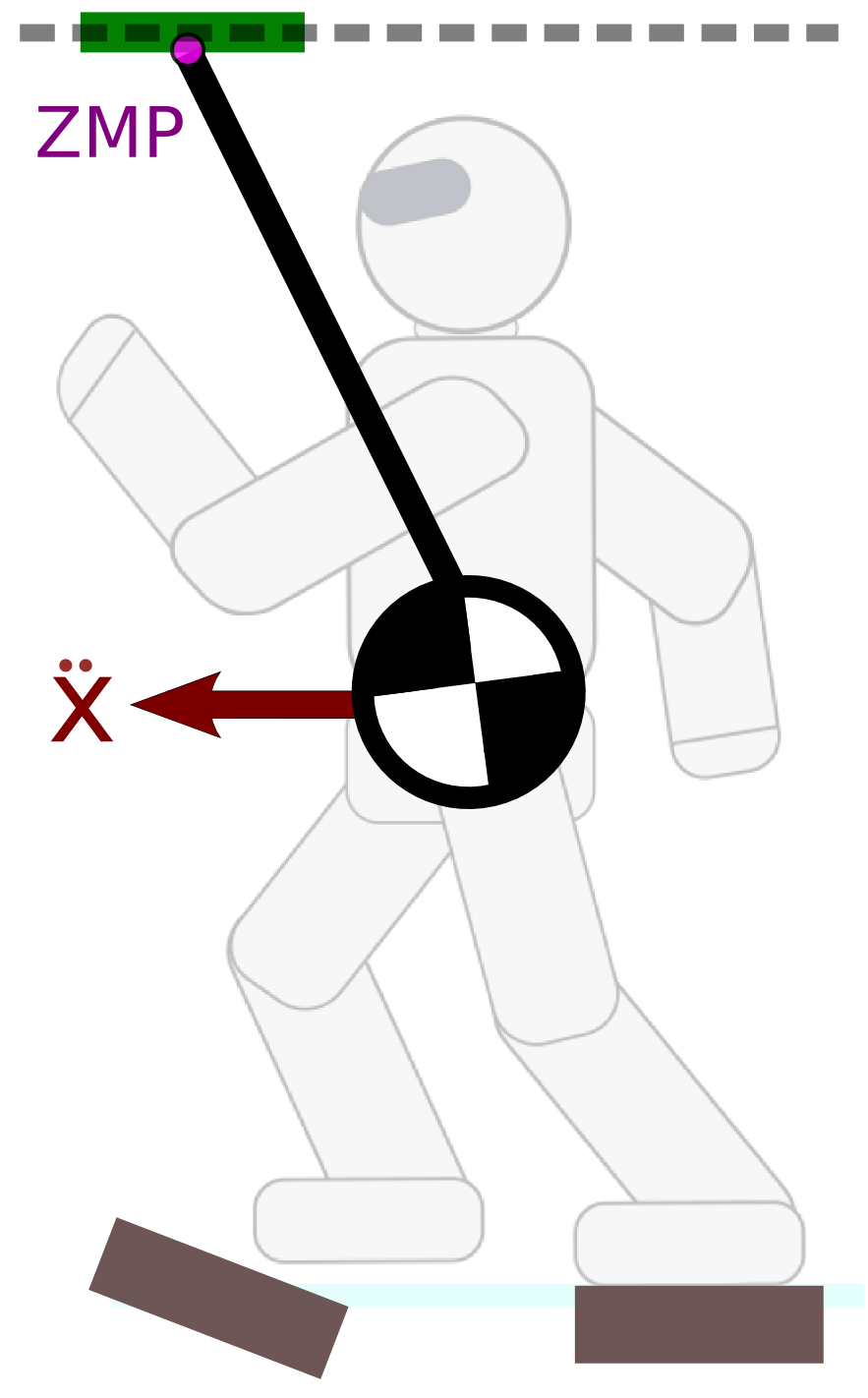# Contact Wrench Cone## Rationale

In multi-contact, frictional constraints are way more critical than torque limits.

## Definition

Friction cone of resultant contact wrench.

## Reduced model

Center of mass + Angular momentum.

## Pipeline

We don't know how to interpolate the angular momentum, therefore:## Discussion

• Whent it fails: update the COM path.
• Can we avoid the IK step for the angular momentum?
(e.g. using the Linear Pendulum Mode where ˙LG=0)

# Reduced-model Support Polytopes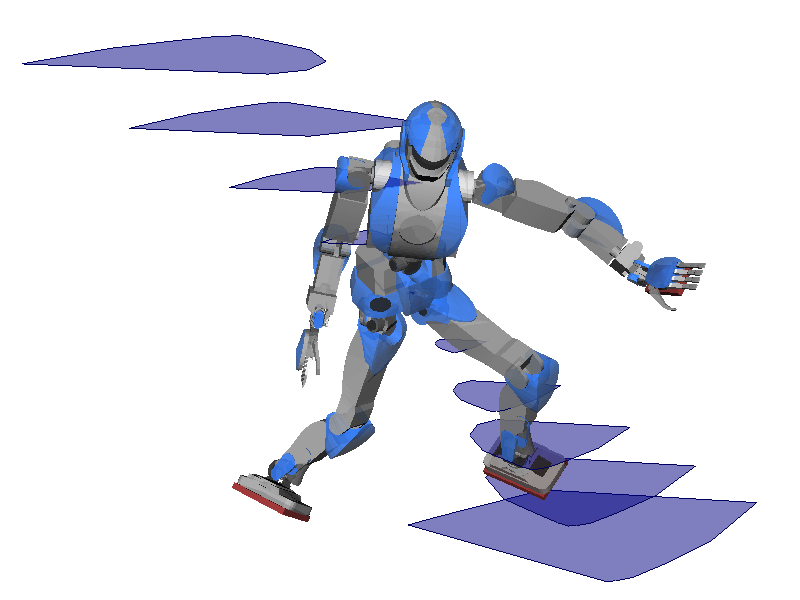Multi-contact ZMP support areas
[Caron et al., TRO 2016]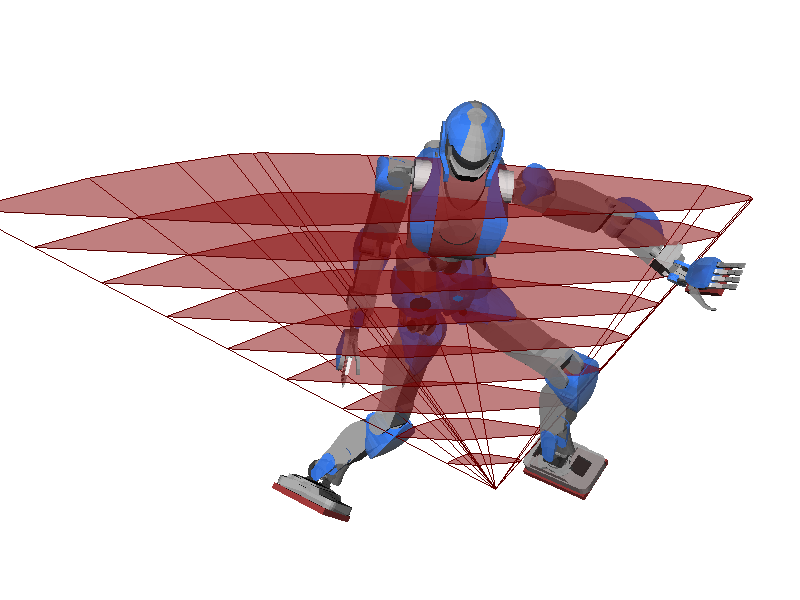COM acceleration support volume
[Caron et al., Humanoids 2016]

# Step timings

Fixed step timings do not work any more in multi-contact, where proper timings depend on terrain topology.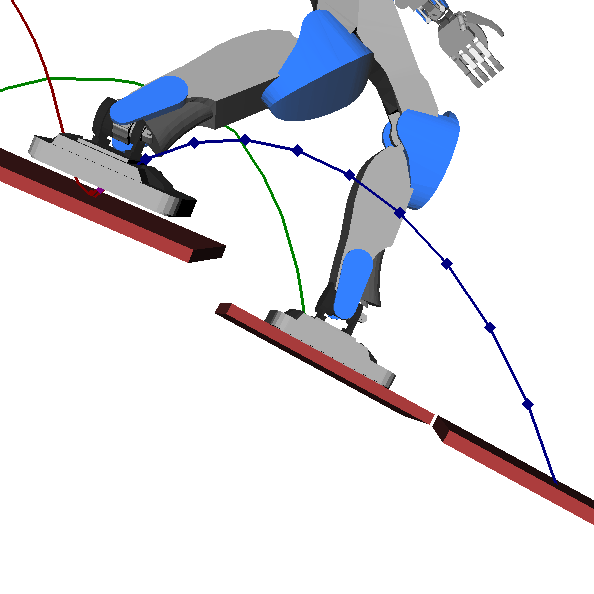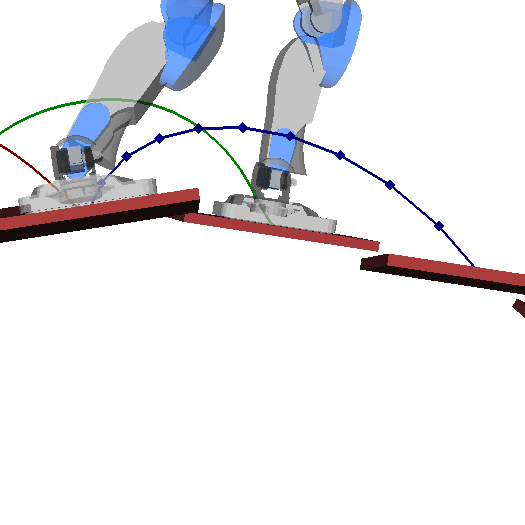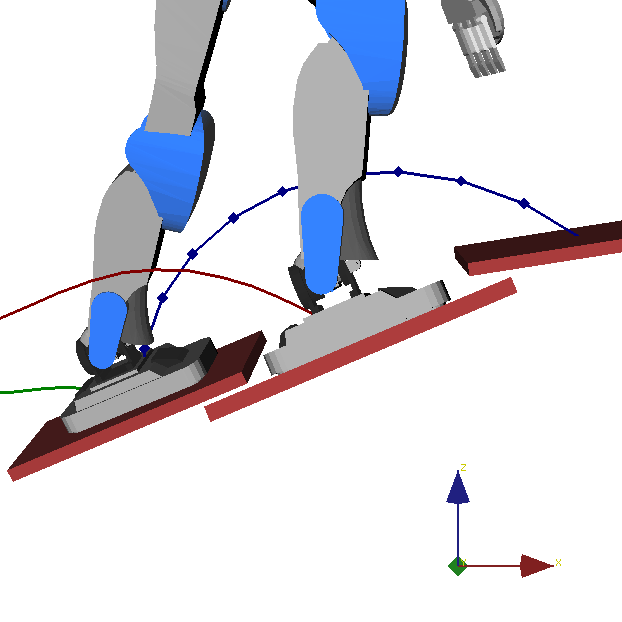# Multi-contact MPC on 3D COM# TOPP-MPC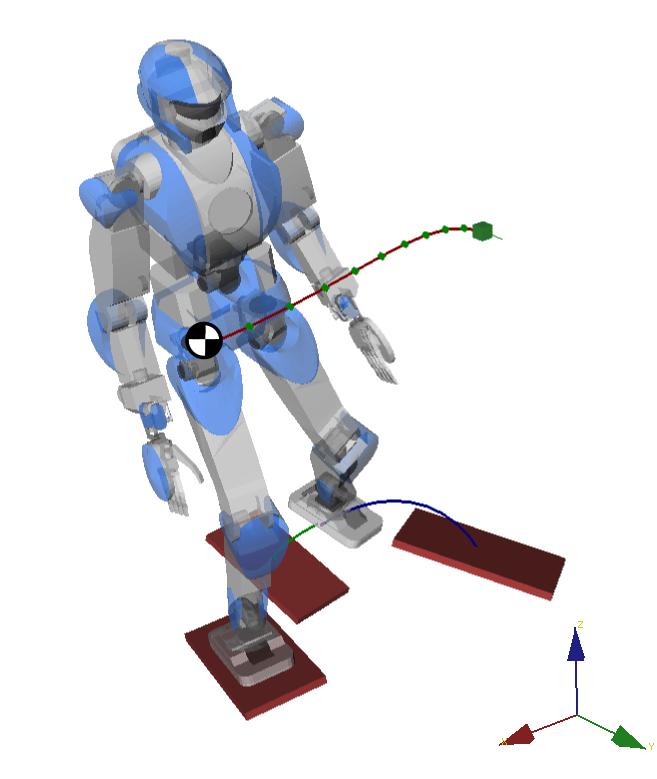## Idea

Use TOPP as dynamics filter in a predictive controller.

## Main benefit

Step timings are computed automatically.

## Contact switch in preview window

Parameterize foot trajectory, feed back step timing to COM optimization.

## PipelineContinuous interpolation/retiming loop running at 20-30 Hz.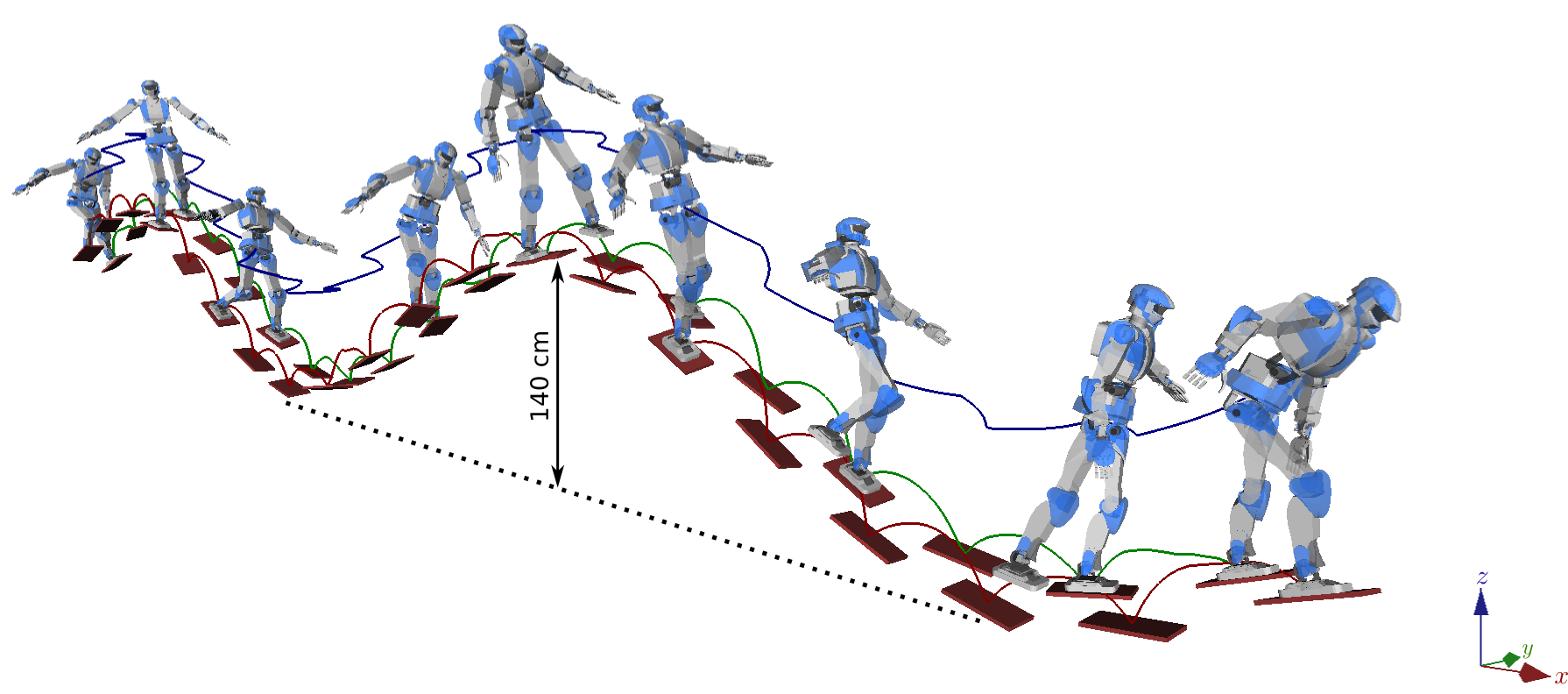https://github.com/stephane-caron/topp-mpc

# Concluding remarks

## Time-optimal retiming

A fast dynamics filter.

## Whole-body TOPP

Core idea of polytope projection: force/torques are not explicitly computed.

## Reduced-model TOPP

Low-dimensional model for replanning and faster computations.

## Predictive control

Nonlinear optimization running at 20-30 Hz in the control loop.